#### Number Sequences 1

What is the 8th:
a) Odd number; 15
b) Square number; 64
c) Prime number. 19

### Factors

Find all the factors of:

34

1, 2, 17, 34.

### Multiples

Subtract the 5th from the 10th multiples of:

5

25

### Polygons

What are the names of regular polygons with:
a) eight sides;
b) nine sides;
c) ten sides.

Octagon, Nonagon and Decagon (all regular)

### Rounding

Round the following numbers to three significant figures:
a) 81.61; 81.6
b) 741514; 742000
c) 0.002995; 0.00300

### Area of a Triangle

Find the area of a triangle that has a base of 7cm and a height of 10cm.

35cm2

### Area of a Trapezium

Find the area of a trapezium that has a base of 9cm, a height of 6cm and a top (parallel to base) of 3cm. 36cm2

Evaluate:

$$\frac{1}{3} + \frac{4}{5}$$ $$= 1\frac{2}{15}$$

### Fractions (Multiplying)

Evaluate:

$$\frac{3}{5} × \frac{7}{8}$$ $$= \frac{21}{40}$$

### Fractions (Dividing)

Evaluate:

$$\frac{3}{5} ÷ \frac{9}{7}$$ $$= \frac{7}{15}$$

### Circle (Vocabulary)

Name the red part.### Venn Diagrams

Describe the red region.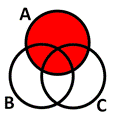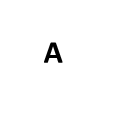### Shape Formulas

What is the formula?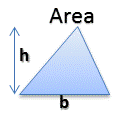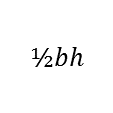What is it?### Fraction to Percentage

Convert this fraction to a percentage.

$$\frac{4}{5}$$ $$= 80$$%

### Circle Area

Find the area of a circle that has a radius of 11cm. Give your answer to three significant figures.

380cm2

### Circle Circumference

Find the circumference of a circle that has a radius of 9cm. Give your answer to three significant figures.

56.5cm2

Calculate the value of:

6.8 + 2.7

= 9.5

### Decimals (Subtracting)

Calculate the value of:

7.2 − 1.7

= 5.5

### Decimals (Multiplying)

Calculate the value of:

4.6 × 3.3

= 15.18

### Decimals (Dividing)

Calculate the value of:

52.8 ÷ 16

= 3.3

### Indices (Simple)

What is the value of:

22

= 4

What is the value of:

5-3

= 0.008

Calculate the value of:

28 + 59

= 87

### Basic Subtraction

Calculate the value of:

72 − 27

= 45

### Basic Multiplication

Calculate the value of:

29 × 72

= 2088

### Basic Division 2

Calculate the value of:

864 ÷ 16

= 54

### Percentage (Of)

Find the value of:

65% of 260

= 169

### Standard Form 1

Find the value of:

5.9 × 103

= 5900

### Highest Common Factor

Find the highest common factor of thirty five and twenty.

= 5

### Times Tables (2-5)

 5 × 5 = 25 7 × 2 = 14 6 × 4 = 24 9 × 5 = 45 4 × 3 = 12 8 × 2 = 16 3 × 5 = 15 2 × 4 = 8

### Times Tables (2-12)

 6 × 4 = 24 5 × 6 = 30 8 × 6 = 48 3 × 6 = 18 7 × 4 = 28 9 × 2 = 18 4 × 4 = 16 2 × 8 = 16

### Times Tables (2)

 9 × 2 = 18 3 × 2 = 6 4 × 2 = 8 7 × 2 = 14 8 × 2 = 16 6 × 2 = 12 5 × 2 = 10 2 × 2 = 4

### Times Tables (3)

 7 × 3 = 21 3 × 3 = 9 5 × 3 = 15 8 × 3 = 24 6 × 3 = 18 9 × 3 = 27 4 × 3 = 12 2 × 3 = 6

### Times Tables (4)

 8 × 4 = 32 6 × 4 = 24 9 × 4 = 36 4 × 4 = 16 5 × 4 = 20 3 × 4 = 12 7 × 4 = 28 2 × 4 = 8

### Times Tables (5)

 9 × 5 = 45 8 × 5 = 40 3 × 5 = 15 5 × 5 = 25 6 × 5 = 30 7 × 5 = 35 4 × 5 = 20 2 × 5 = 10

### Times Tables (6)

 5 × 6 = 30 4 × 6 = 24 8 × 6 = 48 6 × 6 = 36 9 × 6 = 54 3 × 6 = 18 7 × 6 = 42 2 × 6 = 12

### Times Tables (7)

 4 × 7 = 28 6 × 7 = 42 5 × 7 = 35 8 × 7 = 56 3 × 7 = 21 9 × 7 = 63 7 × 7 = 49 2 × 7 = 14

### Times Tables (8)

 5 × 8 = 40 6 × 8 = 48 4 × 8 = 32 8 × 8 = 64 3 × 8 = 24 9 × 8 = 72 7 × 8 = 56 2 × 8 = 16

### Times Tables (9)

 8 × 9 = 72 4 × 9 = 36 5 × 9 = 45 3 × 9 = 27 7 × 9 = 63 9 × 9 = 81 6 × 9 = 54 2 × 9 = 18

### Times Tables (12)

 8 × 12 = 96 6 × 12 = 72 5 × 12 = 60 4 × 12 = 48 7 × 12 = 84 3 × 12 = 36 9 × 12 = 108 2 × 12 = 24

### Fractions (Equivalent)

Write this fraction in its simplest form:

$$\frac{6}{18}$$ $$= \frac{1}{3}$$

### Fractions (Mixed)

Evaluate:

$$2\frac{4}{5} − \frac{8}{9}$$ $$= 1\frac{41}{45}$$

### Pythagoras

Find BC if AB = 5.2m and AC = 7.1m. 8.80m

### Trigonometry (Angle)

Find angle ABC if AB = 3.2m and BC = 4.4m. 43.3o

### Trigonometry (Side)

Find BC if angle BCA = 57o and AC = 5.9m. 10.8m

2

2

2

### Fraction to Decimal

Convert this fraction to a decimal.

$$\frac{3}{4}$$ $$= 0.75$$

### Decimal to Fraction

Convert this decimal to a fraction.

$$0.26$$ = $$\frac{13}{50}$$

### Percentage (Increase)

Increase £60 by 15%

£69

### Lowest Common Multiple

What is the lowest common multiple of six and twenty four.

= 24

### Sequence (Arithmetic)

4,15,26,37,48...

Find the:
a) next term; 59
b) nth term; 11n - 7
c) term number 49; 532

### Sequence (Geometric)

3,6,12,24,48...

Find the:
a) next term; 96
b) nth term; 3 × 2n-1
c) term number 9; 768

### Interest (Simple)

If £200 is invested for 4 years with a simple interest rate of 5%, find the amount of interest earned. £40.00

### Interest (Compound)

If £160 is invested with an interest rate of 3% compounded annually, find the value of the investment after 9 years. £208.76

### Currency Exchange

If £1 is worth $1.59, convert: a) £220 to dollars;$349.80

b) \$120 to pounds; £138.36

### Coordinates (Midpoint)

What are the coordinates of the midpoint of the line joining:

$$(9,7) \text{ and } (17,19)$$

(13,13)

What is the gradient of the line joining:

$$(-8,-1) \text{ and } (-5,5)$$

2

### Coordinates (Square)

Here are the coordinates of 3 vertices of a square, what are the coordinates of the 4th?

$$(4,1),(7,4),(1,4)$$

(4,7)

### Negative Numbers

a) 5 − 13 = -8
b) 5 × (-10) = -50
c) (7−16)(10−17) = 63
d) 50 ÷ (-10) = -5
e) (-11)2 = 121

### Substitution

If p = 5, q = 19 and
r = -12 evaluate:

a) 2q − p = 33
b) pq + r = 83
c) p2 − 5q - r = -58

### Equations (Type 1)

Solve:

$$4x = 36$$

$$x = 9$$

### Equations (Type 2)

Solve:

$$3x +9= 30$$

$$x = 7$$

### Equations (Type 3)

Solve:

$$3x -4= 2x + -2$$

$$x = 2$$

### Equations (Type 4)

Solve:

$$5(2x +4)-7= 63$$

$$x = 5$$

### Equations (Type 5)

Solve:

$$5(3x + 3)= 4(3x + 5)$$

$$x = 1.67 \text{(to 3 sf)}$$

### Equations (Simultaneous 1)

Solve:

$$5x+5y = 35$$
$$4x-5y = 1$$

$$x = 4, y = 3$$

### Equations (Simultaneous 2)

Solve:

$$2x-4y = -2$$
$$5x+8y = 49$$

$$x = 5, y = 3$$

### Equations (Simultaneous 3)

Solve:

$$6x+7y = -96$$
$$6x-3y = -6$$

$$x = -5.5, y = -9$$

### Sets (Union)

Find the union of:

{5,6,7,8,9,10} and
{3,4,5,6,7,8}

{3,4,5,6,7,8,9,10}

### Sets (Intersection)

Find the intersection of:

{5,6,7,8,9,10} and
{2,6,12}

{6}

### Bearings

A plane flies from point A to point B on a bearing of 111o. What bearing would it return on from B to A? 291o

### Probability

A number is picked at random from the set

{2,4,6,8,10}

what is the probability it is even? 1

Evaluate:

5 + (9 × 72 − 8)

438

### Simplify

Simplify the following by collecting like terms:

$$5a−5−4a−5$$

$$a-10$$

### Ratio

Divide 120 in the ratio

8:7

64 and 56

### Graph (Linear)

Draw a rough sketch of the graph of:

$$y=-2x$$

y intercept 0

### Prime Factors

Express the following number as the product of prime numbers:

47

47

### Percentage (Reverse)

In a sale an item costs £65 after a 35% reduction. What was the original price?

£100

### Averages

Find the mean, mode, median and range of the following:

5,3,7,5,10

Mean = 6, mode = 5,
median = 5 and range = 7

### Time (Analogue)

What time is this?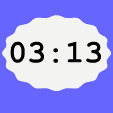### Time (Digital)

Sketch a clock face: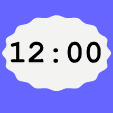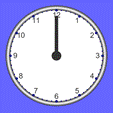### Decimals (Recurring)

Write the following recurring decimal as a fraction in its lowest terms.

0.999999... $$\frac{1}{1}$$

### Percentage (Decrease)

Decrease £40 by 20%

£32

### Brackets (Linear)

Expand:

$$5(3x-8)$$

$$15x-40$$

Expand:

$$(x+4)(4x-3)$$

$$4x^2+13x-12$$

### Factorise (Linear)

Factorise:

$$20x-15$$

$$5(4x-3)$$

Factorise:

$$x^2+2x-3$$

$$(x+3)(x-1)$$

Factorise:

$$5x^2+18x-8$$

$$(x+4)(5x-2)$$

### Circle Theorems

Which theorem?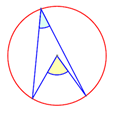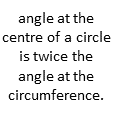### Standard Form 2

Find the value of:

6.35 × 10-5

= 0.0000635

### Standard Form 3

Write in standard form:

4660000

= 4.66 × 106

### Standard Form 4

Write in standard form:

0.0000926

= 9.26 × 10-5

Find the nth term:

$$11, 19, 29, 41, 55,$$

$$n^2+5n+5$$

### Standard Form 5

Multiply 6 × 104
by 5 × 103 and give the answer in standard form.

= 3 × 108

Solve:

$$x^2-x-12= 0$$

$$x = 4$$ and $$-3$$

Solve this equation giving the solutions to 3 significant figures:

$$4x^2-3x-4 = 0$$

$$x = 1.44$$ and $$-0.693$$

### Polygon Angles

What is the size of each exterior angle of a regular decagon?

36°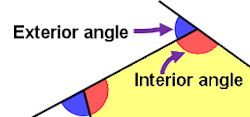### Change The Subject

Make $$d$$ the subject of the formula
$$e=\frac{2d-1}{3}$$

$$d=\frac{3e+1}{2}$$

### Basic Division 1

Calculate the value of:

5481 ÷ 7

= 783

### Number Sequences 2

What is the 12th:
a) Cube number; 1728
b) Triangular number; 78
c) Fibonacci number. 144

### Square Numbers

What are the next three square numbers after
25

36, 49, 64

### Prime Numbers

What are the next three prime numbers after
17

19, 23, 29

### Last Lesson

Write down something you learnt in the previous mathematics lesson.

### Last Week

Write down something you learnt in one of the mathematics lessons last week.

## A Mathematics Lesson Starter Of The Day

Share
• Jan, South Canterbury
•
• Thank you for sharing such a great resource. I was about to try and get together a bank of starters but time is always required elsewhere, so thank you.
• Barbara Schindler, Newton Rigg College
•
• I use Refreshing Revision a lot but today all the brackets and fractions keep coming up 'jumbled' . There are curly brackets, part words / 's in odd places and it is impossible to make out the question. It is doing this on 2 computers I have tried. Can you help? thank you.

[Transum: Sorry to hear about this problem Barbara. I have tested it from here and it seems to be working OK. Please take a look at the MathJax FAQ. Many apologies for the inconvenience.]
• Lesley, UK
•
• Answers for the starter would be great so students can get immediate feedback and become independent learners.

[Thanks for your comments Lesley. The answers are only available to signed-in teachers and parents I'm afraid. I you are a subscriber and are projecting this Starter for the whole class to see you can scroll down the page and show the same questions with the answers included in red.]
• Mrs B, Stockport
•
• Refreshing Revision really useful resource, that I have actually used for Ks2 revision as some topics are appropriate. I'd love to see either ks2 version, with purely ks2 SATs level topics or adding them to the current version.

[Transum: Thanks so much for your feedback Mrs B. If you could send me a list of your top ten ideas for topic you would like to see added I will work on it]
• Mark Adams, St Peters RC School Solihull
•
• It would be great if these questions came with answers as well.

[Transum: The answers to Transum activities are available to those signed in to their Transum subscription account. You can sign up for an account here.]

How did you use this starter? Can you suggest how teachers could present or develop this resource? Do you have any comments? It is always useful to receive feedback and helps make this free resource even more useful for Maths teachers anywhere in the world.

If you don't have the time to provide feedback we'd really appreciate it if you could give this page a score! We are constantly improving and adding to these starters so it would be really helpful to know which ones are most useful. Simply click on a button below:

Excellent, I would like to see more like this
Good, achieved the results I required
Satisfactory
Didn't really capture the interest of the students
Not for me! I wouldn't use this type of activity.

This starter has scored a mean of 3.3 out of 5 based on 516 votes.

Previous Day | This starter is for 9 April | Next Day

## Concept Selection

Tick (or untick) the boxes above to select the concepts you want to be included in this Starter. The display at the top of this page will change instantly to show your choices. You can also drag the panels above so that the questions are ordered to meet your needs.

This Starter is called Refreshing Revision because every time you refresh the page you get different revision questions.

Regularly use this Starter to keep that important learning from being forgotten. Here is the web address (URL) for the version of this page with your currently selected concepts:

Copy and paste the URL above into your lesson plan or scheme of work.

For more ideas on revision there are plenty of tips, suggestions and links on the Mathematics Revision page.

Christmas Present Ideas

It is often very difficult choosing Christmas presents for family and friends but so here are some seasonal, mathematics-related gifts chosen and recommended by Transum Mathematics.

## Equate board game

Here's a great board game that will give any family with school-aged kids hours of worthwhile fun. Christmas is a time for board games but this one will still be useful at any time of year. Games can be adapted to suit many levels of Mathematical ability.

For Maths tutors working with just one or small groups of pupils this game has proved to be an excellent activity for a tutorial. Deciding on the best moves can spark pertinent discussions about mathematical concepts.

Equate looks a bit like Scrabble--for aspiring mathematicians, that is. Designed by a real mathematician, it works like this: You put down tiles on a board and make points by correctly completing simple equations. Your nine tiles include both numbers and mathematical symbols; you can add on to previous plays both vertically and horizontally. more...## How Not To Be Wrong

The maths we learn in school can seem like an abstract set of rules, laid down by the ancients and not to be questioned. In fact, Jordan Ellenberg shows us, maths touches on everything we do, and a little mathematical knowledge reveals the hidden structures that lie beneath the world's messy and chaotic surface. In How Not to be Wrong, Ellenberg explores the mathematician's method of analyzing life, from the everyday to the cosmic, showing us which numbers to defend, which ones to ignore, and when to change the equation entirely. Along the way, he explains calculus in a single page, describes Gödel's theorem using only one-syllable words, and reveals how early you actually need to get to the airport.

What more could the inquisitive adult want for Christmas? This book makes a cosy, interesting read in front of the fire on those cold winter evenings. more...

## Graphic Display Calculator

This handheld device and companion software are designed to generate opportunities for classroom exploration and to promote greater understanding of core concepts in the mathematics and science classroom. TI-Nspire technology has been developed through sound classroom research which shows that "linked multiple representation are crucial in development of conceptual understanding and it is feasible only through use of a technology such as TI-Nspire, which provides simultaneous, dynamically linked representations of graphs, equations, data, and verbal explanations, such that a change in one representation is immediately reflected in the others.

For the young people in your life it is a great investment. Bought as a Christmas present but useful for many years to come as the young person turns into an A-level candidate then works their way through university. more...The analytics show that more and more people are accessing Transum Mathematics via an iPad as it is so portable and responsive. The iPad has so many other uses in addition to solving Transum's puzzles and challenges and it would make an excellent gift for anyone.

The redesigned Retina display is as stunning to look at as it is to touch. It all comes with iOS, the world's most advanced mobile operating system. iPad Pro. Everything you want modern computing to be. more...

## Aristotle's Number Puzzle

It’s a bit of a tradition to give puzzles as Christmas Gifts to nieces and nephews. This puzzle is ideal for the keen puzzle solver who would like a challenge that will continue over the festive period (at least!).

This number puzzle involves nineteen numbers arranged into a hexagon. The goal of the puzzle is to rearrange the numbers so each of the fifteen rows add up to 38. It comes in a wooden style with an antique, aged look.

Keep the Maths in Christmaths with this reasonably priced stocking filler. more...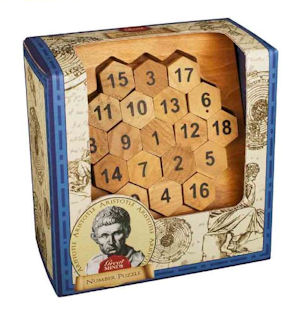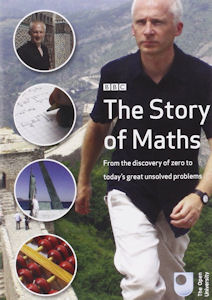## The Story Of Maths [DVD]

The films in this ambitious series offer clear, accessible explanations of important mathematical ideas but are also packed with engaging anecdotes, fascinating biographical details, and pivotal episodes in the lives of the great mathematicians. Engaging, enlightening and entertaining, the series gives viewers new and often surprising insights into the central importance of mathematics, establishing this discipline to be one of humanity s greatest cultural achievements. This DVD contains all four programmes from the BBC series.

Marcus du Sautoy's wonderful programmes make a perfect Christmas gift more...

## Christmas Maths

This book provides a wealth of fun activities with a Christmas theme. Each photocopiable worksheet is matched to the Numeracy Strategy and compatible with the Scottish 5-14 Guidelines. This series is designed for busy teachers in the late Autumn term who are desperate for materials that are relevant and interesting and that can be completed with minimun supervision.

All the activities are suitable for use by class teachers, supply teachers, SEN teachers and classroom assistants and cover topics such as 'How many partridges did the true love give all together?' and 'Filling a sleigh with presents by rolling a dice!'. Children will have lots of fun working through the Christmas Maths themes but also gain valuable skills along the way.

A great source of ideas and another reasonably priced stocking filler. more...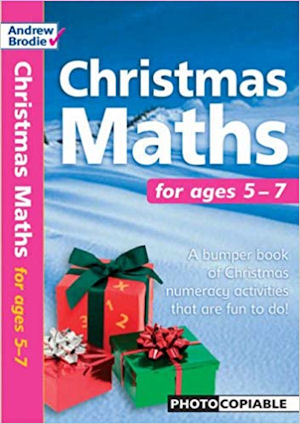## A Compendium Of Mathematical Methods

How many different methods do you know to solve simultaneous equations? To multiply decimals? To find the nth term of a sequence?

A Compendium of Mathematical Methods brings together over one hundred different approaches from classrooms all over the world, giving curious mathematicians the opportunity to explore fascinating methods that they've never before encountered.

If you teach mathematics to any age group in any country, you are guaranteed to learn lots of new things from this delightful book. It will deepen your subject knowledge and enhance your teaching, whatever your existing level of expertise. It will inspire you to explore new approaches with your pupils and provide valuable guidance on explanations and misconceptions. more...

I had been tutoring the wonderful Betsy for five years. When the day came for our last ever session together before the end of her Year 13, I received this beautiful book as a gift of appreciation.

This a very readable book by Ben Orlin. I'm really enjoying the humour in the writing and the drawings are great.

Ben Orlin answers maths' three big questions: Why do I need to learn this? When am I ever going to use it? Why is it so hard? The answers come in various forms-cartoons, drawings, jokes, and the stories and insights of an empathetic teacher who believes that mathematics should belong to everyone.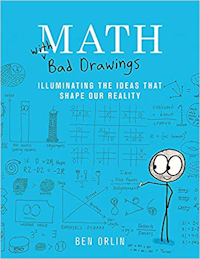Click the images above to see all the details of these gift ideas and to buy them online.

Your access to the majority of the Transum resources continues to be free but you can help support the continued growth of the website by doing your Amazon shopping using the links on this page. Below is an Amazon search box and some items chosen and recommended by Transum Mathematics to get you started.Teacher, do your students have access to computers?Do they have iPads or Laptops in Lessons? Whether your students each have a TabletPC, a Surface or a Mac, this activity lends itself to eLearning (Engaged Learning).Transum.org/go/?Start=April9

Here is the URL which will take them to a related student activity.

Transum.org/go/?to=topictestTeacher:
Scroll down the
page to see how
this Starter can be customised so that it
is just right for